# 网格与圆管层流

• 最近在做一项研究，但是一直被网格非正交困扰。已知条件:1.二维的圆形网格；2.计算z方向的速度分布；3.压力由一维达西公式计算得到，作为源项放入标量输运方程。4.最后可以得到层流的速度分布；由于忽略了U,V方向，因此没有对流项。这是一个稳态有压力源项的椭圆方程。
下图是网格非正交计算结果为：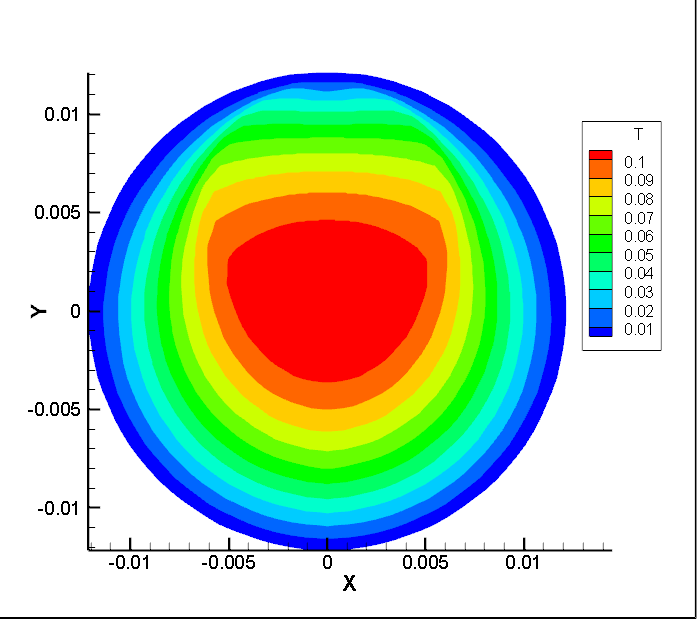正交网格为：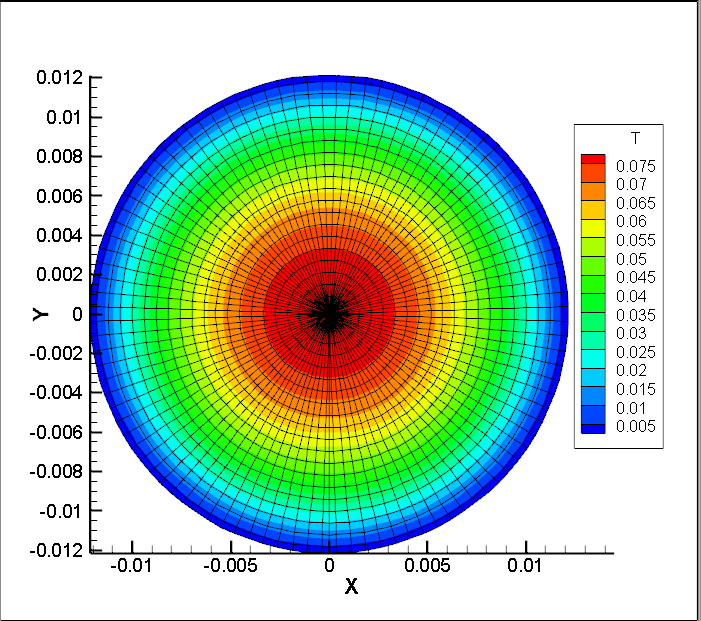正交网格标准计算结果为：关于网格非正交的处理和梯度的处理方式是参考Ferziger, J.H. and M. PeriC, Computational Methods for Fluid Dynamics. 3 ed. 2002, Germany: Springer.第8.6.2的内容，因为书上有示例代码，实现是没有问题的。
希望得到各位CFDer的解答。

• 如果你有方程，可以把方程贴出来，我在openfoam植入并测试

• @东岳
控制方程为：
\begin{equation}
0=-\frac{\partial p}{\partial z}+\mu \left( \frac{{{\partial }^{2}}W}{\partial {{x}^{2}}}+\frac{{{\partial }^{2}}W}{\partial {{y}^{2}}} \right)
\end{equation}
其中：下式结果由达西层流公式计算得到的结果
\begin{equation}
-\frac{\partial p}{\partial z}=2.2
\end{equation}
已知条件：圆管直径为1寸管=0.0243m，中间的小圆直径为大圆的一半=0.01215m，小圆只在中心线上偏心（指的是小圆圆心位置，大圆圆心位置在（0，0）点，小圆（0,y））。我设置的偏心高度y=0.45R（大圆半径）。
介质：水的物性，密度1000，动力粘度0.001；
网格附件：grid1.zip

• 所以你算的就是二维的一个拉普拉斯方程：$\mu\nabla^2 W=2.2$，我觉得你可以添加一下非正交修正看看结果怎么样，类似这种问题很常见（OpenFOAM编程指南里面有一个算例），需要添加非正交修正。另外就是在费正交网格上，测试有无非正交修正的效果，可能已经有效果了但是比较差

• @东岳 这个我添加了非正交修正了，就是上图的这个效果。非正交修正的方法很多，我用了最简单的，基于网格中心的插值延迟修正。• 你可以对比有无非正交修正的效果，第一个图只有有非正交修正的

• @东岳 李老师，这个我已经解决了哈。我一会儿放一下结果。不过偏心率是有要求的不能太高。。。• 这里已经解决了，虽然用的方法精度不是很高，但是非正交修正还是很重要的。梯度计算和扩散项的延迟修正对于计算有很大影响。
1.网格是柱坐标，不采用非正交修正：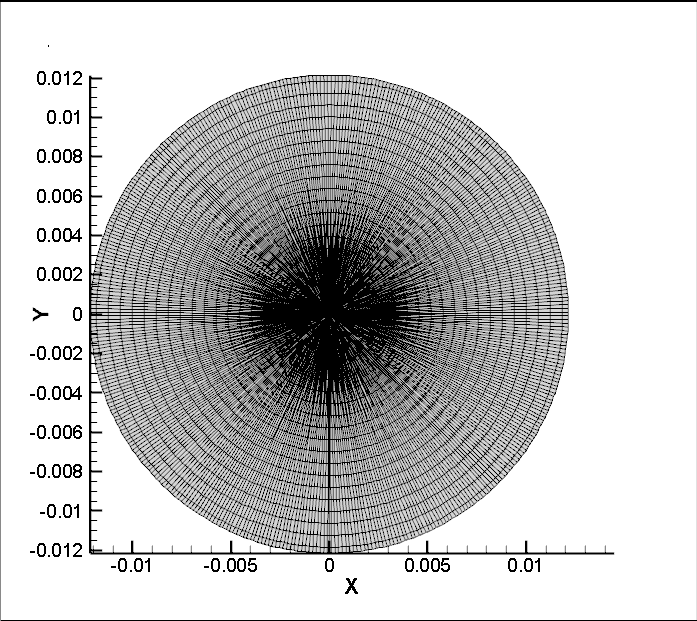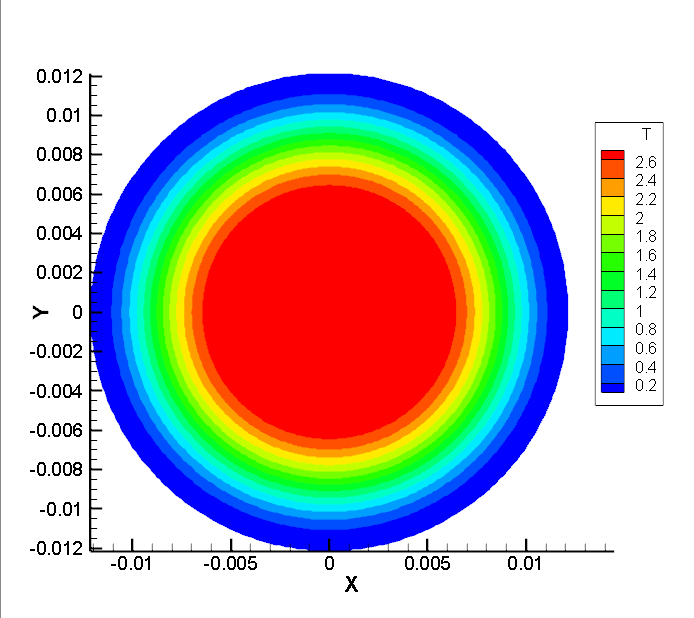可以看出速度场是不对的。
2.网格非正交，不采用非正交修正：这个也是与标准结果不符合！！！
3.网格正交，采用非正交修正：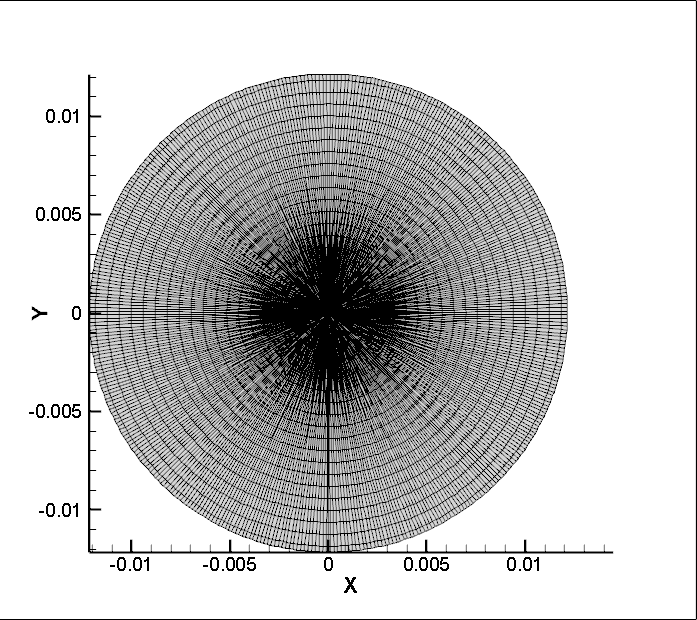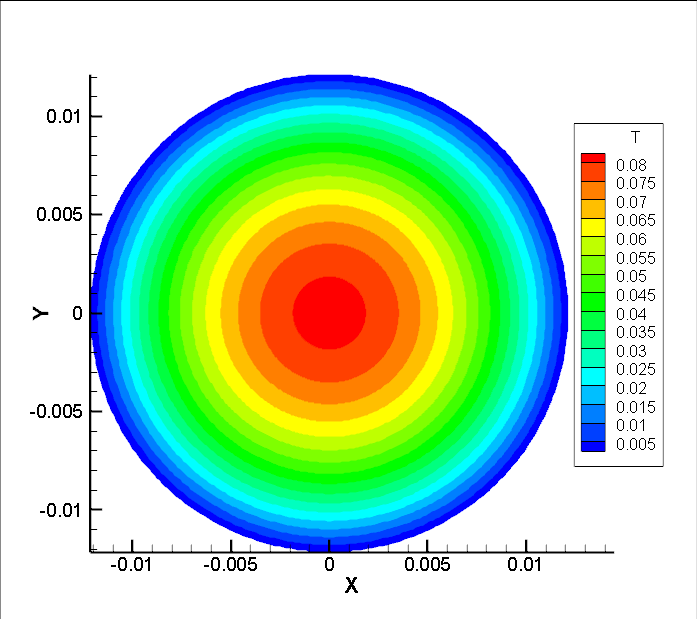计算结果是正确的！
4.网格非正交，采用非正交修正：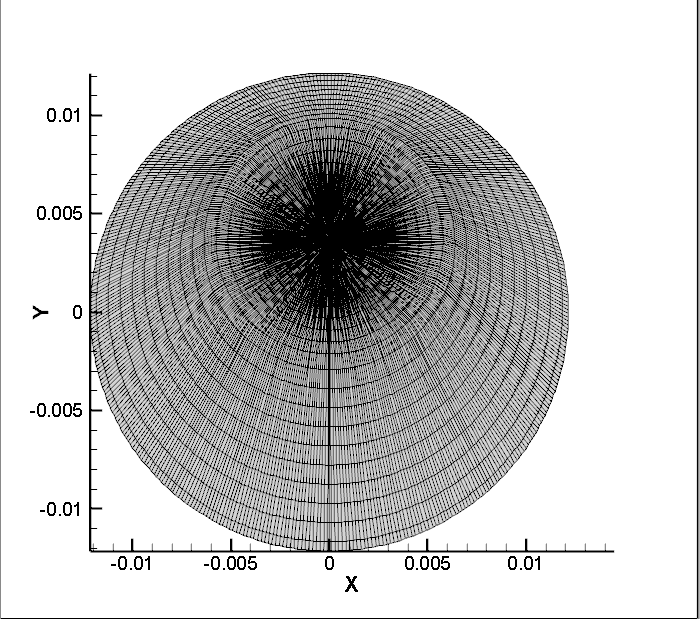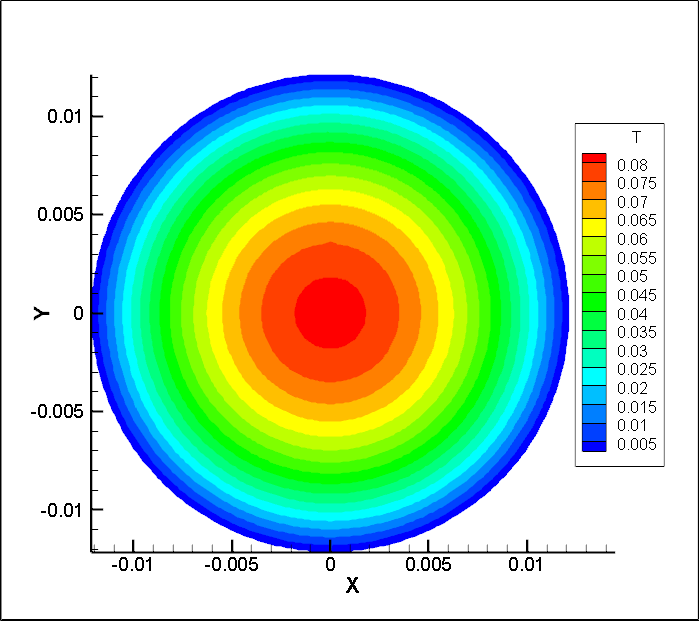计算结果是正确的！
其实网格少点计算也是这样。非正交修正还是非常重要的！！！•excellent

• @东岳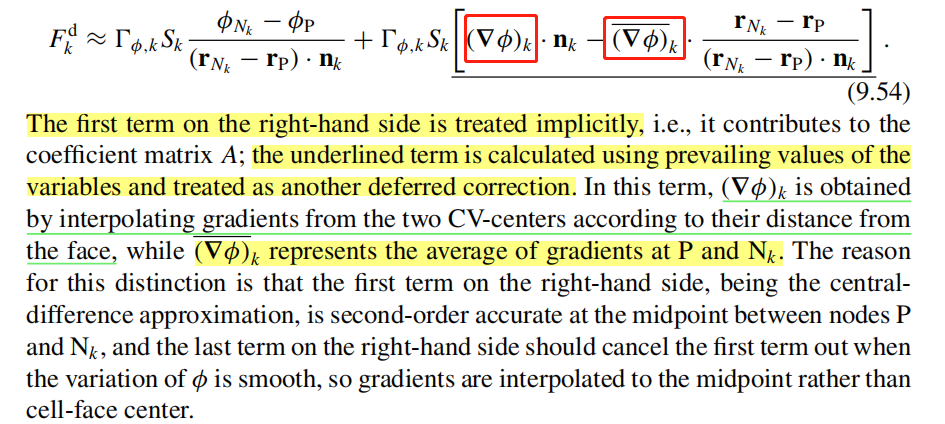想咨询一下东岳老师，这两个梯度的区别，实在不知道怎么计算，谢谢谢谢~

• $\nabla\phi$就是求梯度后向面插值 $\overline{\nabla\phi}$是对当前网格与相邻网格所有网格点的梯度做平均

• @东岳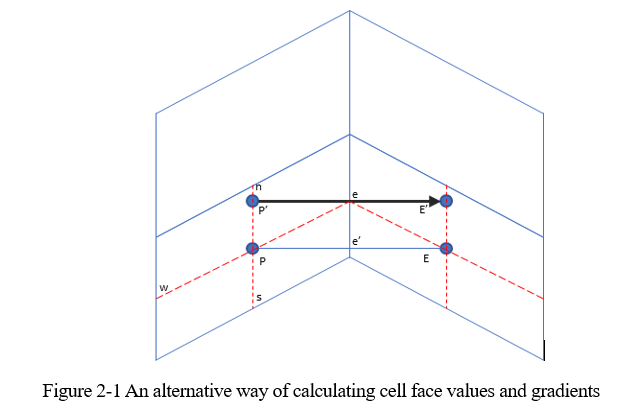差分因子设定为：FX表示的是面上的插值因子，坐标和面相同。
\begin{equation}
FAC=\frac{\left| \overrightarrow{Pe} \right|}{\left| \overrightarrow{Pe} \right|+\left| \overrightarrow{eE} \right|}
\end{equation}
网格P和N格心之间的中间坐标e’计算：
\begin{equation}
{{P}_{{{e}'}}}={{P}_N}\times{FAC}+{{P}_P}\times{FACP}
\end{equation}
网格P和N格心之间的中间梯度计算：
\begin{equation}
\nabla {{\phi }_{e'}}=\nabla {{\phi }_{P}}^{old}FACP+\nabla {{\phi }_{N}}^{old}FAC
\end{equation}
网格P和N中间界面面心坐标e计算：
\begin{equation}
{{P}_{e}}=0.5({{P}_{1}}+{{P}_{2}})
\end{equation}
中间界面值$ϕ_e$的计算：
\begin{align}
& {{\phi }_{{{P}'}}}={{\phi }_{P}}+{{(\nabla \phi )}_{P}}\centerdot (\overrightarrow{{{r}_{P'}}}-\overrightarrow{{{r}_{P}}}) \
& {{\phi }_{e}}={{\phi }_{e'}}+{{(\nabla \phi )}_{e'}}\centerdot (\overrightarrow{{{r}_{e}}}-\overrightarrow{{{r}_{e'}}}) \
\end{align}

\begin{align}
& {{\phi }_{e}}={{\phi }_{E}}\times FAC+{{\phi }_{P}}\times FACP+{{\left( \frac{\partial \phi }{\partial x} \right)}_{e'}}\left( {{x}_{e}}-{{x}_{e'}} \right)+{{\left( \frac{\partial \phi }{\partial y} \right)}_{e'}}\left( {{y}_{e}}-{{y}_{e'}} \right) \
& ={{\phi }_{N}}FAC+{{\phi }_{P}}FACP+\nabla {{\phi }_{{{e}'}}}\bullet \left( \overrightarrow{{{r}_{e}}}-\overrightarrow{{{r}_{e'}}} \right) \
& ={{\phi }_{e'}}+{{(\nabla \phi )}_{e'}}\centerdot (\overrightarrow{{{r}_{e}}}-\overrightarrow{{{r}_{e'}}})
\end{align}

其中：$\overline{\nabla \phi }\text{=}\frac{1}{N}\sum\limits_{i}{\nabla {{\phi }_{i}},i=P,E,W,N,S}$,东岳老师这个是这样算吗？参考文献：
On the discretization of the diffusion term in finite-volume continuum mechanics
Numerical method for coupled fluid flow, heat transfer and stress analysis using unstructured moving meshes with cells of arbitrary topology
Ferziger J H, PeriC M.Computational Methods for Fluid Dynamics. 3. Germany: Springer,2002.
Ferziger J H, PeriC M.Computational Methods for Fluid Dynamics. 4. Germany: Springer,2020.

• @东岳 岳哥，帮忙看看呗，我写的公式是否正确，特别是梯度的算数平均那里哈~

• 公事太多了我晕了• @东岳主要是这个哈

• 文中说it represents the average of gradients at P and N_k，所以我理解是这样的

• @东岳 谢谢东岳老师哈